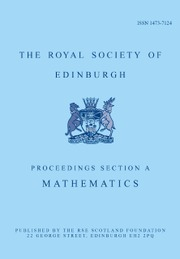Home
Hostname: page-component-768dbb666b-qc52z Total loading time: 0.884 Render date: 2023-02-03T14:01:57.434Z Has data issue: true Feature Flags: { "useRatesEcommerce": false } hasContentIssue trueProceedings of the Royal Society of Edinburgh Section A: Mathematics

# On a backward problem for nonlinear time fractional wave equations

Published online by Cambridge University Press:  24 November 2021

## Abstract

In this paper, we concern with a backward problem for a nonlinear time fractional wave equation in a bounded domain. By applying the properties of Mittag-Leffler functions and the method of eigenvalue expansion, we establish some results about the existence and uniqueness of the mild solutions of the proposed problem based on the compact technique. Due to the ill-posedness of backward problem in the sense of Hadamard, a general filter regularization method is utilized to approximate the solution and further we prove the convergence rate for the regularized solutions.

Type
Research Article
Information

## Access options

Get access to the full version of this content by using one of the access options below. (Log in options will check for institutional or personal access. Content may require purchase if you do not have access.)

## References

Alvarez, E., Gal, C., Keyantuo, V. and Warma, M.. Well-posedness results for a class of semi-linear super-diffusive equations. Nonlinear Anal. 181 (2019), 2461.CrossRefGoogle Scholar
Chen, D., Hofmann, B. and Zou, J.. Regularization and convergence for ill-posed backward evolution equations in Banach spaces. J. Differ. Equ. 265 (2018), 35333566.CrossRefGoogle Scholar
He, J. W. and Peng, L.. Approximate controllability for a class of fractional stochastic wave equations. Comput. Math. Appl. 78 (2019), 14631476.CrossRefGoogle Scholar
Huang, J., Wang, G. and Xiong, J.. A maximum principle for partial information backward stochastic control problems with applications. SIAM J. Control. Optim. 48 (2009), 21062117.CrossRefGoogle Scholar
Huynh, L. N., Zhou, Y., O'Regan, D. and Tuan, N. H.. Fractional Landweber method for an initial inverse problem for time-fractional wave equations. Appl. Anal 100 (2021), 860878.CrossRefGoogle Scholar
Jia, J., Peng, J., Gao, J. and Li, Y.. Backward problem for a time-space fractional diffusion equation. Inverse Probl. Imaging 12 (2018), 773800.CrossRefGoogle Scholar
Kilbas, A. A., Srivastava, H. M. and Trujillo, J. J.. Theory and applications of fractional differential equations, vol. 204, pp. 323 (Amsterdam: Elsevier Science B.V., 2006).CrossRefGoogle Scholar
Kim, I., Kim, K. H. and Lim, S.. An $L_q(L_p)$-theory for the time fractional evolution equations with variable coefficients. Adv. Math. 306 (2017), 123176.CrossRefGoogle Scholar
Li, Y., Wang, Y. and Deng, W.. Galerkin finite element approximations for stochastic space–time fractional wave equations. SIAM J. Numer. Anal. 55 (2017), 31733202.CrossRefGoogle Scholar
Mainardi, F.. Fractional relaxation-oscillation and fractional diffusion-wave phenomena. Chaos, Solitons Fractals 7 (1996), 14611477.CrossRefGoogle Scholar
Mainardi, F.. Fractional calculus and waves in linear viscoelasticity, an introduction to mathematical models (London: Imperial College Press, 2010).CrossRefGoogle Scholar
Mainardi, F. and Paradisi, P.. A model of diffusive waves in viscoelasticity based on fractional calculus. Proc. 36th IEEE Conf. Decis. Control 5 (1997), 49614966.CrossRefGoogle Scholar
Otárola, E. and Salgado, A. J.. Regularity of solutions to space–time fractional wave equations: a PDE approach. Fract. Calc. Appl. Anal. 21 (2018), 12621293.CrossRefGoogle Scholar
Peng, L. and Huang, Y.. On nonlocal backward problems for fractional stochastic diffusion equations. Comput. Math. Appl. 78 (2019), 14501462.CrossRefGoogle Scholar
Sakamoto, K. and Yamamoto, M.. Initial value/boundary value problems for fractional diffusion-wave equations and applications to some inverse problems. J. Math. Anal. Appl. 382 (2011), 426447.CrossRefGoogle Scholar
Tuan, N. H., Ao, V., Huynh, L. N. and Zhou, Y.. Regularization of a backward problem for the inhomogeneous time-fractional wave equation. Math. Method. Appl. Sci. 43 (2020), 54505463.CrossRefGoogle Scholar
Tuan, N. H., Debbouche, A. and Ngoc, T. B.. Existence and regularity of final value problems for time fractional wave equations. Comput. Math. Appl. 78 (2019), 13961414.CrossRefGoogle Scholar
Tuan, N. H., Huynh, L. N., Ngoc, T. B. and Zhou, Y.. On a backward problem for nonlinear fractional diffusion equations. Appl. Math. Lett. 92 (2019), 7684.CrossRefGoogle Scholar
Wang, J., Wei, T. and Zhou, Y.. Optimal error bound and simplified Tikhonov regularization method for a backward problem for the time-fractional diffusion equation. J. Comput. Appl. Math. 279 (2015), 277292.CrossRefGoogle Scholar
Wei, T. and Wang, J.. A modified quasi-boundary value method for the backward time-fractional diffusion problem. ESAIM: Math. Model. Numer. Anal. 48 (2014), 603621.CrossRefGoogle Scholar
Wei, T. and Zhang, Y.. The backward problem for a time-fractional diffusion-wave equation in a bounded domain. Comput. Math. Appl. 75 (2018), 36323648.CrossRefGoogle Scholar
Zhou, Y.. Basic theory of fractional fifferential equations (Singapore: World Scientific, 2014).CrossRefGoogle Scholar
Zhou, Y., He, J. W., Ahmad, B. and Tuan, N. H.. Existence and regularity results of a backward problem for fractional diffusion equations. Math. Meth. Appl. Sci. 42 (2019), 67756790.CrossRefGoogle Scholar
2
Cited by

# Save article to Kindle

Note you can select to save to either the @free.kindle.com or @kindle.com variations. ‘@free.kindle.com’ emails are free but can only be saved to your device when it is connected to wi-fi. ‘@kindle.com’ emails can be delivered even when you are not connected to wi-fi, but note that service fees apply.

Find out more about the Kindle Personal Document Service.

On a backward problem for nonlinear time fractional wave equations
Available formats
×

# Save article to Dropbox

To save this article to your Dropbox account, please select one or more formats and confirm that you agree to abide by our usage policies. If this is the first time you used this feature, you will be asked to authorise Cambridge Core to connect with your Dropbox account. Find out more about saving content to Dropbox.

On a backward problem for nonlinear time fractional wave equations
Available formats
×

# Save article to Google Drive

To save this article to your Google Drive account, please select one or more formats and confirm that you agree to abide by our usage policies. If this is the first time you used this feature, you will be asked to authorise Cambridge Core to connect with your Google Drive account. Find out more about saving content to Google Drive.

On a backward problem for nonlinear time fractional wave equations
Available formats
×
×